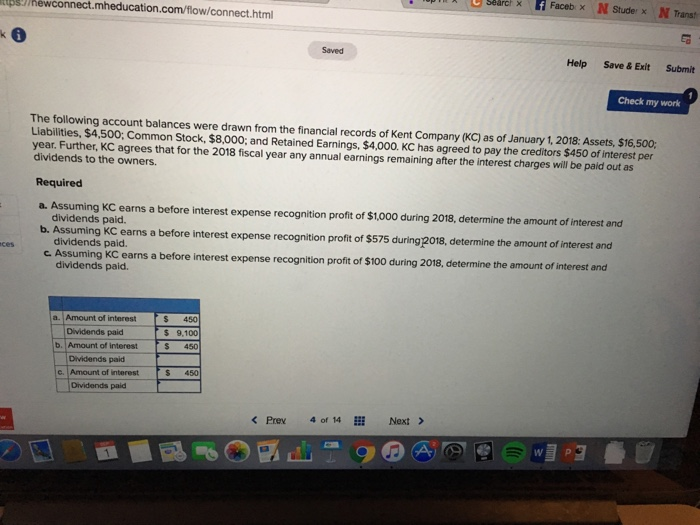# Question & Answer: The following account balances were drawn from the financial records of Kent Company (KC) as of January 1,2018:…..The following account balances were drawn from the financial records of Kent Company (KC) as of January 1,2018: Assets, \$16,500: Liabilities, \$4,500: Common Stock, \$8,000: and Retained Earnings, \$4,000. KC has agreed to pay the creditors \$450 of interest per year. Further, KC agrees that for the 2018 fiscal year any annual earnings remaining after the interest charges will be paid out as dividends to the owners. a. Assuming KC earns a before interest expense recognition profit of \$1,000 during 2018, determine the amount of interest and dividends paid. b. Assuming KC earns a before interest expense recognition profit of \$575 during 2018, determine the amount of interest and dividends paid. c. Assuming KC earns a before interest expense recognition profit of \$100 during 2018, determine the amount of interest and dividends paid.

a.

Don't use plagiarized sources. Get Your Custom Essay on
Question & Answer: The following account balances were drawn from the financial records of Kent Company (KC) as of January 1,2018:…..
GET AN ESSAY WRITTEN FOR YOU FROM AS LOW AS \$13/PAGE

Amount of interest = \$450

Dividends paid = \$1,000 – \$450 = \$550

b.

Amount of interest = \$450

Dividends paid = \$575 – \$450 = \$125

c.

Amount of interest = \$450

Dividends paid = \$0